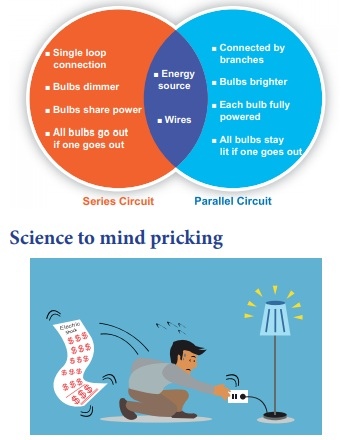Home | | Science 7th Std | Electric circuit and its Types

# Electric circuit and its Types

In a cell, the longer line denotes the positive (+) terminal and the short line denotes the negative (-) terminal. We shall use these symbols to show components in the circuits we draw. Such diagrams are called circuit diagrams.

Electric circuit

It is difficult to draw a realistic diagram of this circuit. The electrical appliances you use at home have even more difficult circuits. Can you draw realistic diagrams of such circuits which contain many bulbs, cells, switches and other components? Do you think it is easy? It is not easy.

Scientists have tried to make the job easier. They have adopted simple symbols for different components in a circuit. We can draw circuit diagrams using these symbols.

Symbols for bulbs, cells and switches are shown in figure.In a cell, the longer line denotes the positive (+) terminal and the short line denotes the negative (-) terminal. We shall use these symbols to show components in the circuits we draw. Such diagrams are called circuit diagrams.

All muscles of our bodies move in response to electrical  impulses generated naturally in our bodiesTypes of electrical circuits

In the above experiment, we make a circuit with a bulb and a cell. We make only one kind of the circuit with a cell and a bulb. But we can make many types of circuits if we have more than one bulb or cells by connecting these components in different ways .

1. Series circuit

Two kinds of circuits can be made with two bulbs and a cell. In this experiment we shall make one of them and study it.

Look at the circuit with two bulbs, and a cell and a switch given here (Figure)It is clear from the circuit diagram, that the two bulbs are connected one after the other. The circuit diagram shows the sequence of the bulbs and cell, not their real position. The way in which the bulbs have been connected in this circuit is called series connection.

Now make the circuit by joining the two bulbs and cell. Do both the bulbs light up? Do both glow equally bright? If one glows less bright, will it shine more brightly if we change its place in the sequence? Change the sequence of bulbs and notice.

Sometimes bulbs appear to be similar can differ from each other. So, similar looking bulb do not always glow equally bright when connected in series. The circuit can be broken at several places. For example, between the cell and the bulb, between the two bulbs etc.

2. Parallel Circuit

Figure - shows a circuit in which two bulbs are connected in different places. This is a second type of circuit. Two bulbs in this circuit are said to be connected in parallel and such circuits are called parallel circuits.Similarity and Difference between Series and Parallel CircuitScience to mind pricking

If an electrician attending an electrical fault at your home gets current shock, will you touch him in order to get rid off him from current risk? Will you use the wet stick to beat him to avoid further effects of electric shock?

Why do the electric line man are wear rubber gloves in their hands while doing electrical works on a electrical pole?

We know that all materials are made up of the basic building block, the ‘atom’. An atom, in turn, contains electrically charged particles. Many of these particles are fixed to the atoms but in conductors (such as all metals) there are lots of particles that are not held to any particular atom but are free to wander around randomly in the metal. These are called ‘free charge’.

Short circuit

You might have observed the spark in the electric pole located nearby your house. Do you know the cause of this electric spark? This is due to the short circuiting of electricity along its path. A short circuit is simply a low resistance connection between the two conductors supplying electrical power to any circuit. Arc welding is a common example of the practical application of the heating due to a short circuit.Tags : Electricity | Term 2 Unit 2 | 7th Science , 7th Science : Term 2 Unit 2 : Electricity
Study Material, Lecturing Notes, Assignment, Reference, Wiki description explanation, brief detail
7th Science : Term 2 Unit 2 : Electricity : Electric circuit and its Types | Electricity | Term 2 Unit 2 | 7th Science